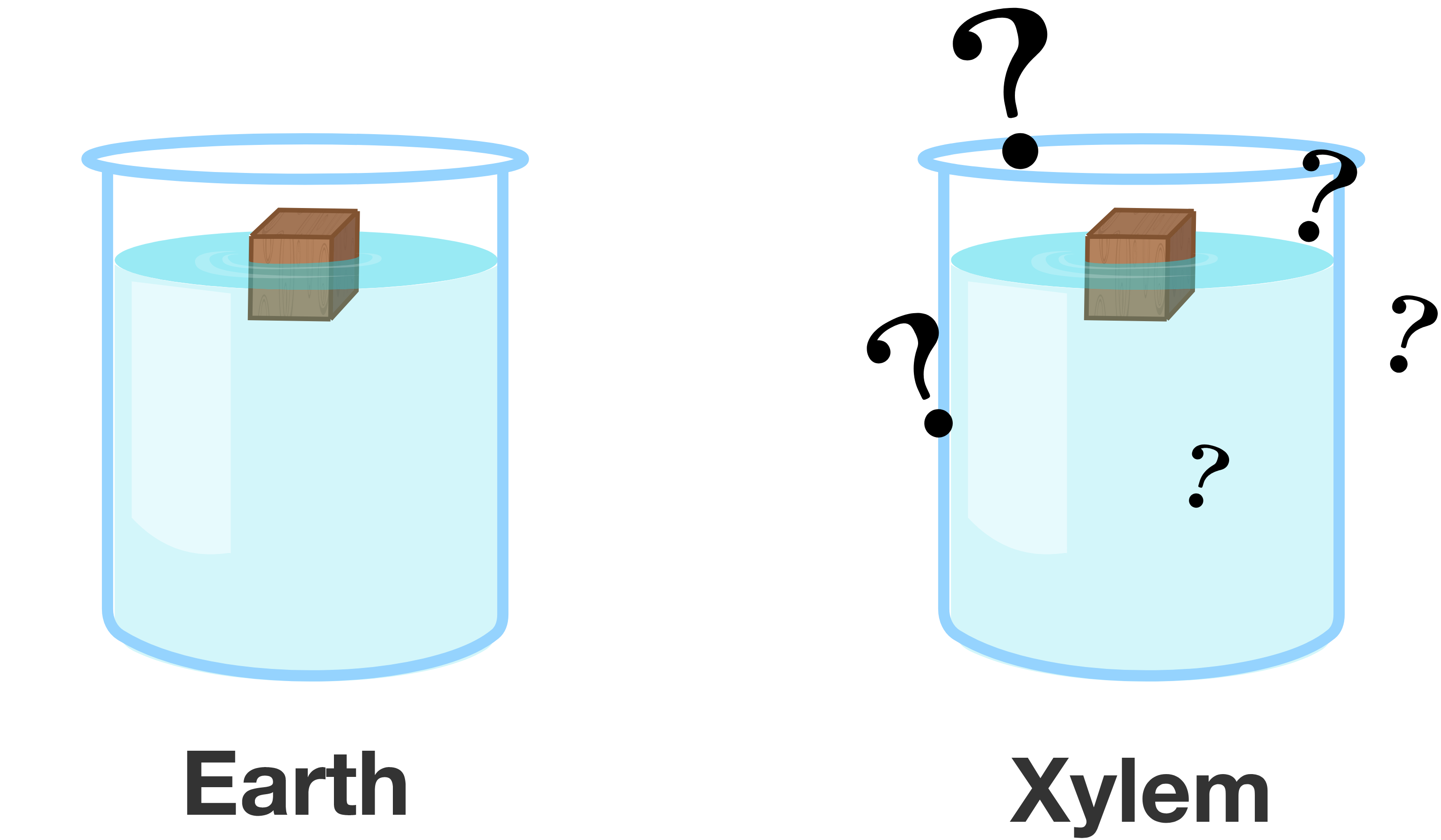# Interplanetary Displacement

A small wooden block floats in a beaker of liquid on Earth, where gravitational acceleration is roughly $\SI[per-mode=symbol]{9.8}{\meter\per\second\squared}.$ An identical wooden block floats in an identical beaker of liquid on planet Xylem, where gravitational acceleration is roughly $\SI[per-mode=symbol]{11.1}{\meter\per\second\squared}.$

How would the volume of displaced water vary in these two scenarios?×